Describing motion along a line

This free course is available to start right now. Review the full course description and key learning outcomes and create an account and enrol if you want a free statement of participation.

Free course

# Derived functions and derivative notation

Given the function x(t) that describes some particular motion, you could plot the corresponding position-time graph, measure its gradient at a variety of times to find the instantaneous velocity at those times and then plot the velocity-time graph. If you had some time left, you might go on to measure the gradient of the velocity-time graph at various times, and then plot the acceleration-time graph for the motion. This would effectively complete the description of the motion, but it would be enormously time consuming and, given the difficulty of reading graphs, not particularly accurate.

Fortunately this graphical procedure can usually be entirely avoided. Starting again from the function x(t), there exists a mathematical procedure, called differentiation, that makes it possible to determine the velocity vx(t) directly, by algebra alone. We shall not try to describe the principles that underpin differentiation, but we will introduce the notation of the subject and list some of the basic results. To make this introduction as general as possible we shall initially consider a general function f(y) rather than the position function x(t).

The central idea is this:

Remember, the gradient of a graph at a given point is defined by the gradient of its tangent at that point.

Given a function f(y) it is often possible to determine a related function of y, called the derived function, with the property that, at each value of y, the derived function is equal to the gradient of the graph of f against y at that same value of y.

The derived function is usually referred to as the derivative of f with respect to y (often abbreviated to derivative) and may be represented by the symbol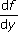or, more formally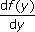. Thenotation is reminiscent of the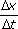notation that was used when discussing the gradient of a straight line and thus provides a clear reminder of the link between the derived function and the gradient of the f against y graph. However, it is important to remember thatis a single symbol representing the derived function, it is not the ratio of two quantities df and dy.

Although there are systematic ways of finding derived functions from first principles, you will not be required to use them in this course. Indeed, physicists are rarely required to do this because tables of derivatives already exist for all the well-known functions, and derivatives of more complicated functions can usually be expressed as combinations of those basic derivatives. Table 6 lists a few of the basic derivatives along with the simplest of the rules for combining them - it also gives some explicit examples of functions and their derivatives. Computer packages are now available that implement the rules of differentiation, these are often used to determine the derivatives of more complicated functions (Figure 32).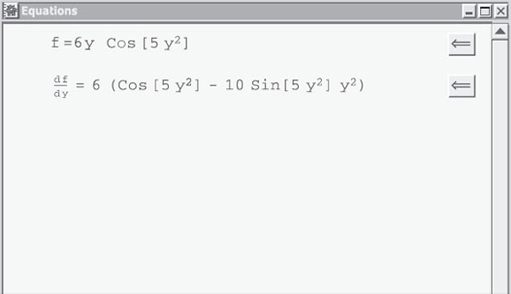Figure 32: A function and its derivative, as displayed by an algebraic computing package

## Table 6: Some simple derivatives. The functions f, g and h depend on the variable y. The quantities A and n are constants, which may be positive, negative or zero. Note that n is not necessarily an integerFunction f(y)Derivative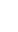Example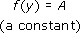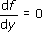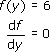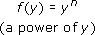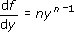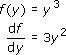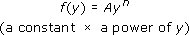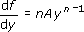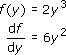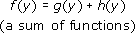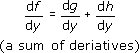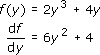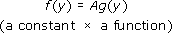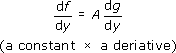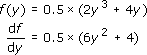The idea of a derivative may be new to you and, if so, may seem rather strange. However, if you know the explicit form of a function, then there are several crucial advantages in using derivatives to determine gradients, rather than making measurements on a graph. In particular, looking up the derivative of a function in a table should be completely accurate, whereas measuring the gradient of the tangent to a graph is always approximate. For example, if f﻿(y﻿) = y﻿2 then the derivative of f﻿(﻿y﻿) is d﻿f﻿/﻿d﻿y = 2y and evaluating the derivative at y = 3 to find the gradient at that particular value of y gives 6. This is an exact result that could not have been obtained with such precision from measurements on a graph. Moreover, if we want to know the gradient at many different values of y, all we need to do is to substitute each of those values into the general expression for the derivative, df/dy = 2y. This is much simpler than drawing many different tangents and measuring their individual gradients.

S207_2

### Take your learning further

Making the decision to study can be a big step, which is why you'll want a trusted University. The Open University has 50 years’ experience delivering flexible learning and 170,000 students are studying with us right now. Take a look at all Open University courses.

If you are new to university level study, find out more about the types of qualifications we offer, including our entry level Access courses and Certificates.

Not ready for University study then browse over 900 free courses on OpenLearn and sign up to our newsletter to hear about new free courses as they are released.

Every year, thousands of students decide to study with The Open University. With over 120 qualifications, we’ve got the right course for you.

Request an Open University prospectus